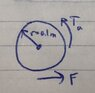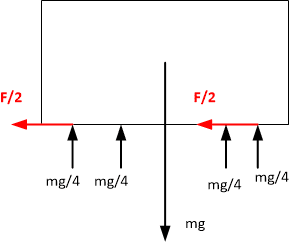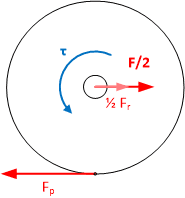# Need help calculating load torque and acceleration torque of a vehicle

• Automotive
chessguy103
Hi everyone,

I'm working on designing a vehicle that uses electric motors, but I'm having a little trouble finding the load and acceleration torques in order to select the right motors to use.

Variables:
m = total mass of vehicle
a = acceleration
μ_r = rolling friction coefficient
N = normal force (vehicle weight)
J = moment of inertia
F_r = rolling friction
T_a = acceleration torque
α = angular acceleration

Assumptions:
• m = 50 kg (distributed evenly across 4 wheels)
• a = 2 m/s^2
• μ_r = 0.02
• r = 0.1 m
• Vehicle driven by two back wheels using two separate electric motors (direct drive)
• Driving on a flat surface

Calculations:
Here's what I've calculated so far. If I've made any mistakes or left anything out, please correct me.

• F_r = μ_r * N
• N = 50 kg * 9.81 m/s^2 = 490.5 N
• F_r = 0.02 * 490.5 N = 9.81 N
• T_L = F_r * r
• T_L = 9.81 N * 0.1 m = 0.981 Nm

Acceleration torque:
I tried calculating this in two ways and got two different answers.

1. First I used a free body diagram, attached below.
• F = ma = 50 kg * 2 m/s^2 = 100 N
• 2 Driving wheels --> 2T = Fd = 100 N*0.1 m = 10 Nm
• T = 5 Nm (per driving wheel)

2. Here's where I'm getting confused.

• T_a = J*α ------- for one wheel
• α = a/r = 2/0.1 = 20 rad/s
• J = 0.5*m*r^2 = 0.5*25*0.1^2 = 0.125 kg*m^2--------------- I used 25 kg here since there are two driving wheels --> each one will accelerate half of the mass, if that makes sense. Is this assumption correct?
• T_a = J*α = 0.125*20 = 2.5 = 1/2 the T_a I previously calculated. What am I doing wrong here?

Any help is appreciated. Thanks :)

#### Attachments

•File_000 (2).jpeg
47.9 KB · Views: 121
Last edited:

Homework Helper
hello @chessguy103 ,!
but I'm having a little trouble
You are doing quite well. A clear list of variables and parameters. Working posted and good to follow. An example for a lot of first posters !

A few improvements:

free body diagram
You do calculations for the body of the vehicle and for the wheels. It is sensible to have two free body diagrams. The one for the body is almost trivial: two driving wheels each exert a forward force that causes the acceleration. Upward force from the four wheels compensates the weight ##mg##.So simple it can be omitted: We don't worry about the vertical forces or the non-driving wheels (but add their friction force at the driving wheels).

This allows us to draw a better FBD for the driving wheels: Yours is a nice start, but it is incomplete. If I leave out the vertical forces (mg/4 up and mg/4 down):There is only one driving torque and it has to provide the acceleration and compensation for the friction, from the driving wheel plus the non-driven wheel, hence ½ Fr.

2. Here's where I'm getting confused.

• T_a = J*α ------- for one wheel
• α = a/r = 2/0.1 = 20 rad/s
• J = 0.5*m*r^2 = 0.5*25*0.1^2 = 0.125 kg*m^2--------------- I used 25 kg here since there are two driving wheels --> each one will accelerate half of the mass, if that makes sense. Is this assumption correct?
• T_a = J*α = 0.125*20 = 2.5 = 1/2 the T_a I previously calculated. What am I doing wrong here?

You found F/2 = 50 N so the torque for acceleration is 5 N##\cdot##m per driving wheel.
The torque to overcome friction has to be added to this, so 0.98/2 N##\cdot##m per driving wheel.

The torque to make the wheels spin should also be added to this, but for that you need the mass of a wheel.
Inserting half the total mass in the moment of inertia is not right: you don't want the whole thing to spin!

With e.g. a wheel mass ##m_w = ## 1 kg you have ##I = {1\over 2} m_w\, r^2## kgm2 per wheel, so 0.02 kgm2 per driving wheel. With ##\alpha = a/r = ## 20 rad/s 2 ##\quad \tau = I\alpha## yields 0.2 N##\cdot##m per driving wheel. So a small correction.

The torque to overcome another type of friction: wind resistance, should still be added to this: depending on the speed to be reached it can be the main torque!

Clear so far ?

##\ ##

••chessguy103, anorlunda and berkeman
Homework Helper
Gold Member
It seems to me that the given value of coeficient of friction, if for contact areas of wheels and flat surface, is excessively low, compared to practical values.
Also, if designing a real vehicle that uses electric motors, for torque calculation, you will need to consider the maximum slope of the roads to travel, as well as to reserve certain safety marging (abnormal loading of the vehicle, high winds, to start movement overcoming a stone on the road, etc.).

•hutchphd and chessguy103
chessguy103
The torque to overcome another type of friction: wind resistance, should still be added to this: depending on the speed to be reached it can be the main torque!

Clear so far ?

##\ ##

Yes, that makes sense! Thank you!

I'm ignoring wind resistance for now since it'll only go up to 20 mph (~9 m/s), and I also don't know the shape of the body yet.

It seems to me that the given value of coeficient of friction, if for contact areas of wheels and flat surface, is excessively low, compared to practical values.
Also, if designing a real vehicle that uses electric motors, for torque calculation, you will need to consider the maximum slope of the roads to travel, as well as to reserve certain safety marging (abnormal loading of the vehicle, high winds, to start movement overcoming a stone on the road, etc.).

μ_r = 0.02 is what I found for rolling friction coefficient between a car tire and asphalt.
And yes, I'm also adding mgsinθ*r for the extra torque needed for an incline. I just wanted to get the main calculations down first. I will also be adding a margin of safety. Thanks :)

•BvU
Homework Helper
I'm ignoring wind resistance for now since it'll only go up to 20 mph (~9 m/s), and I also don't know the shape of the body yet.
As a cyclist, I am very much aware that there is a lot of wind resistance, even at 20 mphAs a dutchman, I know that headwinds can easily double the power required ...

And as a murphist: there seems to exist a conservation law for headwind -- you turn, the wind turns too !##\ ##

••hutchphd and chessguy103
chessguy103
I see. I should account for that as well then.

One other thing: let's assume a coefficient of static friction of μ_s = 0.72 (car tire on asphalt)

That would mean that static friction force for all 4 wheels, F_s = N * μ_s = 50 * 9,81 * 0.72 = 353.16 N

Which means that F_s for a single wheel would be 353.16 / 4 = 88.29 N. So the force at the driving wheels shouldn't exceed this value or else they'll slip. Or, since it's two driving wheels would we do 353.16 / 2?

I'm thinking its the first one since the actual weight/normal force is distributed across all 4 wheels. But I'm not sure.

Homework Helper
Gold Member
μ_r = 0.02 is what I found for rolling friction coefficient between a car tire and asphalt.
And yes, I'm also adding mgsinθ*r for the extra torque needed for an incline. I just wanted to get the main calculations down first. I will also be adding a margin of safety. Thanks :)
One thing is the added resistance to rolling due to the continuos deformation of a rubber tire while rolling over asphalt; another thing is the "traction" or resistance to sliding or skidding of that same tire.

https://www.engineeringtoolbox.com/rolling-friction-resistance-d_1303.html

https://www.engineeringtoolbox.com/friction-coefficients-d_778.html

The second one is what determines how much tangential force your wheels can achieve during acceleration and braking, and its magnitude depends on the normal force and a higher value of static coeficient of friction, which is normally around 0.8 for rubber/dry asphalt.

Welcome to PF!•chessguy103
Homework Helper
I'm thinking its the first one since the actual weight/normal force is distributed across all 4 wheels
I agree: the normal force per wheel is mg/4 and the maximum friction force is therefore ##\mu##mg/4 per wheel, diving or not.

We had another thread on this where a difference between front wheel drive and rear wheel drive was discussed. It starts about hill climbing, but that turns into acceleration (physically more or less equivalent).

##\ ##

•chessguy103
•